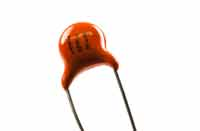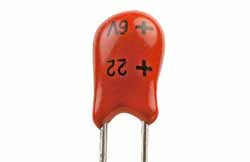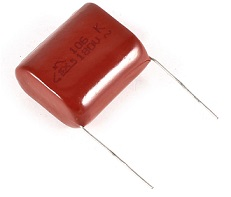# CAPACITOR

## What Is A Capacitor?

capacitor (also called condenser, which is the older term) is an electronic device that stores electric energy. It is similar to a battery, but can be smaller, lightweight and a capacitor charges or discharges much quicker. Capacitors are used in many electronic devices today, and can be made out of many different types of material.Capacitors come in all shapes and sizes, but they usually have the same basic components. There are the two conductors (known as plates, largely for historic reasons) and there’s the insulator in between them (called the dielectric). The two plates inside a capacitor are wired to two electrical connections on the outside called terminals, which are like thin metal legs you can hook into an electric circuit.

### Types of Capacitors

Capacitors are divided in to two common groups:

1. Fixed Capacitor with fixed capacitance values
2. Variable Capacitors with variable (trimmer) or adjustable (tunable) capacitance values.

Out of these the most important group is fixed capacitors. Many capacitors got their names from the dielectric. But this is not true for all capacitors because some old electrolytic capacitors are named by its cathode construction. So the most used names are simply historical.

Fixed capacitors include polarized and non-polarized. Ceramic and Film capacitors are examples of  non-polarized capacitors. Electrolytic and Super Capacitors are included in the group of polarized capacitors.

The classification of fixed capacitors are shown in the figure below. Some of the important capacitors are listed here.

• Ceramic capacitors
• Film and paper capacitors
• Aluminum, tantalum and niobium electrolytic capacitors
• Polymer capacitors
• Supercapacitor
• Silver mica, glass, silicon, air-gap and vacuum capacitor

### Overview of Different Types of Capacitors

As we explained above, there are many different types of capacitor that can be used. Some of the major types are outlined below:

#### Ceramic capacitor:The ceramic capacitor is a type of capacitor that is used in many applications from audio to RF.

Values range from a few picofarads to around 0.1 microfarads. Ceramic capacitor types are by far the most commonly used type of capacitor being cheap and reliable and their loss factor is particularly low although this is dependent on the exact dielectric in use.

In view of their constructional properties, these capacitors are widely used both in leaded and surface mount formats.

#### Electrolytic capacitor:Electrolytic capacitors are a type of capacitor that is polarised.

They are able to offer high capacitance values – typically above 1μF, and are most widely used for low frequency applications – power supplies, decoupling and audio coupling applications as they have a frequency limit if around 100 kHz.

#### Tantalum capacitor:Like electrolytic capacitors, tantalum capacitors are also polarised and offer a very high capacitance level for their volume.

However this type of capacitor is very intolerant of being reverse biased, often exploding when placed under stress. This type of capacitor must also not be subject to high ripple currents or voltages above their working voltage.

They are available in both leaded and surface mount formats.

#### Silver Mica Capacitor:

Silver mica capacitors are not as widely used these days, but they still offer very high levels ofstability, low loss and accuracy where space is not an issue.

They are primarily used for RF applications and and they are limited to maximum values of 1000 pF or so.

#### Polystyrene Film Capacitor:Polystyrene capacitors are a relatively cheap form of capacitor but offer a close tolerance capacitor where needed.

They are tubular in shape resulting from the fact that the plate / dielectric sandwich is rolled together, but this adds inductance limiting their frequency response to a few hundred kHz.

They are generally only available as leaded electronics components.

#### Polyester Film Capacitor:Polyester film capacitors are used where cost is a consideration as they do not offer a high tolerance.

Many polyester film capacitors have a tolerance of 5% or 10%, which is adequate for many applications.
They are generally only available as leaded electronics components.

#### Metallised Polyester Film Capacitor:This type of capacitor is a essentially a form of polyester film capacitor where the polyester films themselves are metallised.

The advantage of using this process is that because their electrodes are thin, the overall capacitor can be contained within a relatively small package.

The metallised polyester film capacitors are generally only available as leaded electronics                                                                    components.

#### Polycarbonate capacitor:The polycarbonate capacitors has been used in applications where reliability and performance are critical.
The polycarbonate film is very stable and enables high tolerance capacitors to be made which will hold their capacitance value over time. In addition they have a low dissipation factor, and they remain stable over a wide temperature range, many being specified from -55°C to +125°C.
However the manufacture of polycarbonate dielectric has ceased and their production is now very limited.

#### Polypropylene Capacitor:The polypropylene capacitor is sometimes used when a higher tolerance type of capacitor is necessary than polyester capacitors offer.
As the name implies, this capacitor uses a polypropylene film for the dielectric. One of the advantages of the capacitor is that there is very little change of capacitance with time and voltage applied.
This type of capacitor is also used for low frequencies, with 100 kHz or so being the upper limit. They are generally only available as leaded electronics components.

#### Glass capacitors:As the name implies, this capacitor type uses glass as the dielectric.

Although expensive, these capacitors offer very high levels or performance in terms of extremely low loss, high RF current capability, no piezo-electric noise and other features making them ideal for many performance RF applications.

#### Supercap:Also known as a supercapacitor or ultracapacitor, as the name implies these capacitors have very large values of capacitance, of up to several thousand Farads.

They find uses for providing a memory hold-up supply and also within automotive applications.

The voltage ( Vc ) connected across all the capacitors that are connected in parallel is THE SAME. Then, Capacitors in Parallel have a “common voltage” supply across them giving:

VC1 = VC2 = VC3 = VAB = 12V

In the following circuit the capacitors, C1C2 and C3 are all connected together in a parallel branch between points A and B as shown.### Capacitors in Parallel

When capacitors are connected together in parallel the total or equivalent capacitance, CT in the circuit is equal to the sum of all the individual capacitors added together. This is because the top plate of capacitor, C1 is connected to the top plate of C2 which is connected to the top plate of C3 and so on.

The same is also true of the capacitors bottom plates. Then it is the same as if the three sets of plates were touching each other and equal to one large single plate thereby increasing the effective plate area in m2.

Since capacitance, C is related to plate area ( C = ε A/d ) the capacitance value of the combination will also increase. Then the total capacitance value of the capacitors connected together in parallel is actually calculated by adding the plate area together. In other words, the total capacitance is equal to the sum of all the individual capacitance’s in parallel. You may have noticed that the total capacitance of parallel capacitors is found in the same way as the total resistance of series resistors.

The currents flowing through each capacitor and as we saw in the previous tutorial are related to the voltage. Then by applying Kirchoff’s Current Law, ( KCL ) to the above circuit, we haveand this can be re-written as:Then we can define the total or equivalent circuit capacitance, CT as being the sum of all the individual capacitance’s add together giving us the generalized equation of:

### Parallel Capacitors EquationWhen adding together capacitors in parallel, they must all be converted to the same capacitance units, whether it is uFnF or pF. Also, we can see that the current flowing through the total capacitance value, CT is the same as the total circuit current, iT

We can also define the total capacitance of the parallel circuit from the total stored coulomb charge using the Q = CV equation for charge on a capacitors plates. The total charge QT stored on all the plates equals the sum of the individual stored charges on each capacitor therefore,As the voltage, ( V ) is common for parallel connected capacitors, we can divide both sides of the above equation through by the voltage leaving just the capacitance and by simply adding together the value of the individual capacitances gives the total capacitance, CT. Also, this equation is not dependent upon the number of Capacitors in Parallel in the branch, and can therefore be generalized for any number of N parallel capacitors connected together.

### Capacitors in Series

For series connected capacitors, the charging current ( iC ) flowing through the capacitors is THE SAME for all capacitors as it only has one path to follow.

Then, Capacitors in Series all have the same current flowing through them as iT = i1 = i2 = i3 etc. Therefore each capacitor will store the same amount of electrical charge, Q on its plates regardless of its capacitance. This is because the charge stored by a plate of any one capacitor must have come from the plate of its adjacent capacitor. Therefore, capacitors connected together in series must have the same charge.

QT = Q1 = Q2 = Q3 ….etc

Consider the following circuit in which the three capacitors, C1C2 and C3  are all connected together in a series branch across a supply voltage between points A and B.

### Capacitors in a Series ConnectionIn the previous parallel circuit we saw that the total capacitance, CT of the circuit was equal to the sum of all the individual capacitors added together. In a series connected circuit however, the total or equivalent capacitance CT is calculated differently.

In the series circuit above the right hand plate of the first capacitor, C1 is connected to the left hand plate of the second capacitor, C2 whose right hand plate is connected to the left hand plate of the third capacitor, C3. Then this series connection means that in a DC connected circuit, capacitor C2 is effectively isolated from the circuit.

The result of this is that the effective plate area has decreased to the smallest individual capacitance connected in the series chain. Therefore the voltage drop across each capacitor will be different depending upon the values of the individual capacitance’s.

Then by applying Kirchoff’s Voltage Law, ( KVL ) to the above circuit, we get:Since Q = CV and rearranging for V = Q/C, substituting Q/C for each capacitor voltage VCin the above KVL equation will give us:dividing each term through by Q gives

### Series Capacitors EquationWhen adding together Capacitors in Series, the reciprocal ( 1/C ) of the individual capacitors are all added together ( just like resistors in parallel ) instead of the capacitance’s themselves. Then the total value for capacitors in series equals the reciprocal of the sum of the reciprocals of the individual capacitances.